Get inspired by the success stories of our students in IIT JAM MS, ISI  MStat, CMI MSc DS.  Learn More

# Intertwined Conditional Probability | ISI MStat 2016 PSB Problem 4This is an interesting problem from intertwined conditional probability and Bernoulli random variable mixture, which gives a sweet and sour taste to Problem 4 of ISI MStat 2016 PSB.

## Problem

Let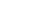and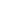be three Bernoulli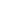random variables such that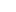and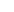are independent,andare independent, andandare independent.
(a) Show that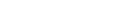.
(b) Show that if equality holds in (a), then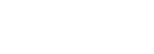### Prerequisites

• Principle of Inclusion and Exclusion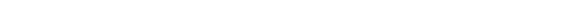• Basic Probability Theory
• Conditional Probability
•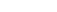iff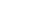or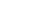or.
•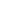= or;= and

## Solution

#### (a)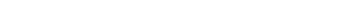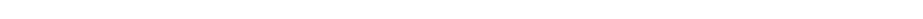We use the fact thatandare independent,andare independent, andandare independent.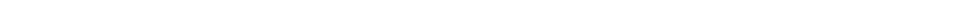.andbe three Bernoullirandom variables. Hence,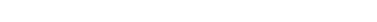.

#### (b)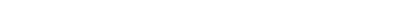.

Now, this is just a logical game with conditional probability.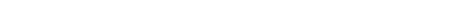...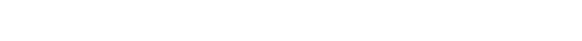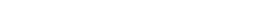.

Now,is a Bernoullirandom variable. So,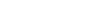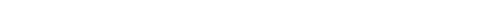.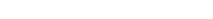....

Hence,.

This is an interesting problem from intertwined conditional probability and Bernoulli random variable mixture, which gives a sweet and sour taste to Problem 4 of ISI MStat 2016 PSB.

## Problem

Letandbe three Bernoullirandom variables such thatandare independent,andare independent, andandare independent.
(a) Show that.
(b) Show that if equality holds in (a), then### Prerequisites

• Principle of Inclusion and Exclusion• Basic Probability Theory
• Conditional Probability
•ifforor.
•= or;= and

## Solution

#### (a)We use the fact thatandare independent,andare independent, andandare independent..andbe three Bernoullirandom variables. Hence,.

#### (b).

Now, this is just a logical game with conditional probability.....

Now,is a Bernoullirandom variable. So,.....

Hence,.

This site uses Akismet to reduce spam. Learn how your comment data is processed.

### Knowledge Partner